# NCERT Solution Class 10 Maths Chapter 7 – Coordinate Geometry

## Exercise 7.1 Class 10 Maths NCERT Solution Page No: 161

1. Find the distance between the following pairs of points:

(i) (2, 3), (4, 1)

(ii) (-5, 7), (-1, 3)

(iii) (a, b), (- a, – b)

Solution:

Distance formula to find the distance between two points (x1, y1) and (x2, y2) is, say d,

2. Find the distance between the points (0, 0) and (36, 15). Can you now find the distance between the two towns A and B discussed in Section 7.2.

Solution:

Let us consider, town A at point (0, 0). Therefore, town B will be at point (36, 15).

Distance between points (0, 0) and (36, 15)

The distance between town A and B will be 39 km.

3. Determine if the points (1, 5), (2, 3) and (-2, -11) are collinear.

Solution: The sum of the lengths of any two line segments is equal to the length of the third line segment then all three points are collinear.

Consider, A = (1, 5) B = (2, 3) and C = (-2, -11)

Find the distance between points; say AB, BC and CA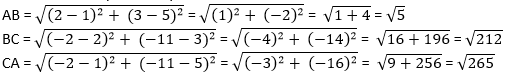Since AB + BC ≠ CA

Therefore, the points (1, 5), (2, 3), and ( – 2, – 11) are not collinear.

4. Check whether (5, – 2), (6, 4) and (7, – 2) are the vertices of an isosceles triangle.

Solution:

Since two sides of any isosceles triangle are equal. To check whether given points are vertices of an isosceles triangle, we will find the distance between all the points.

Let the points (5, – 2), (6, 4), and (7, – 2) are representing the vertices A, B, and C respectively.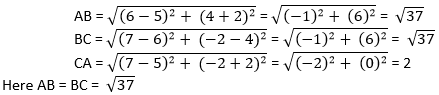This implies, whether given points are vertices of an isosceles triangle.

5. In a classroom, 4 friends are seated at the points A, B, C and D as shown in Fig. 7.8. Champa and Chameli walk into the class and after observing for a few minutes Champa asks Chameli, “Don’t you think ABCD is a square?” Chameli disagrees. Using distance formula, find which of them is correct.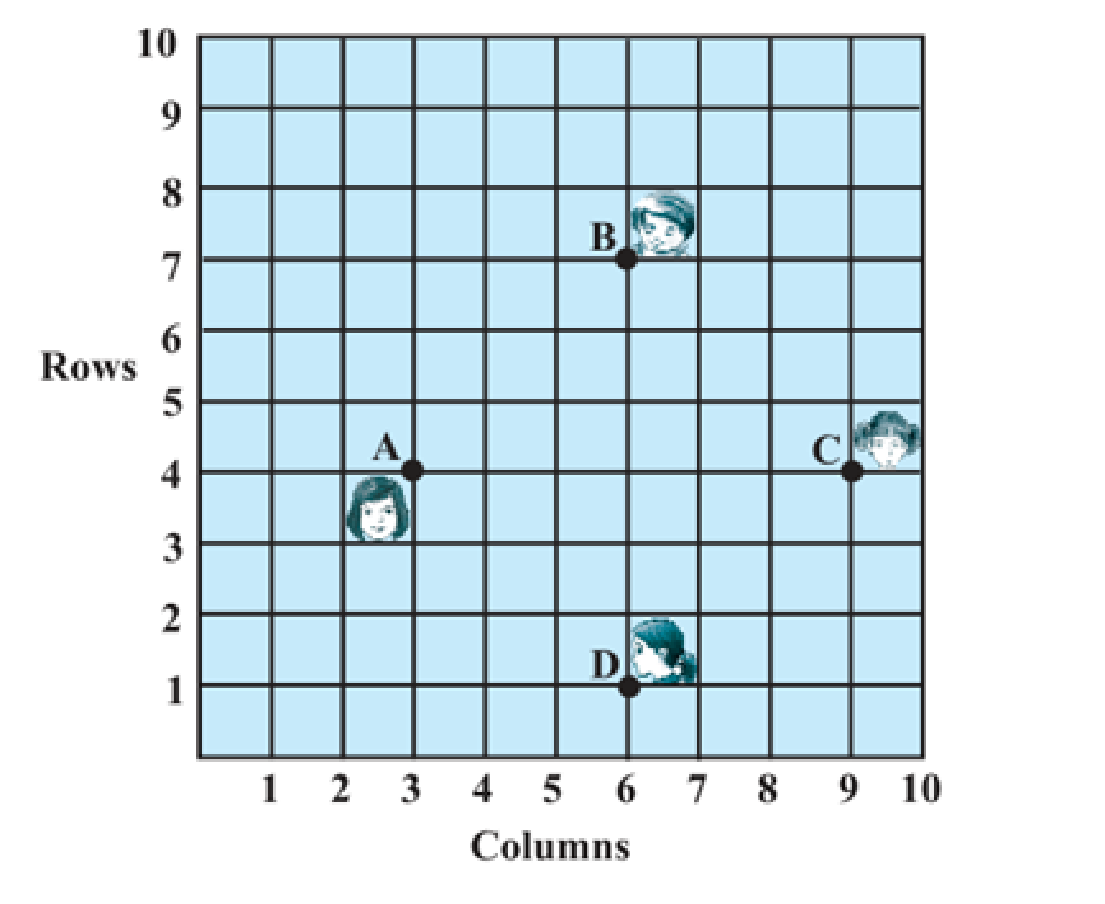Solution:

From figure, the coordinates of points A, B, C and D are (3, 4), (6, 7), (9, 4) and (6,1).

Find distance between points using distance formula, we get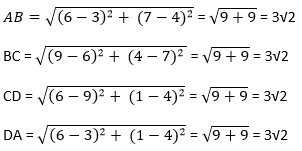AB = BC = CD = DA = 3√2

All sides are of equal length. Therefore, ABCD is a square and hence, Champa was correct.

6. Name the type of quadrilateral formed, if any, by the following points, and give reasons for your answer:

(i) (- 1, – 2), (1, 0), (- 1, 2), (- 3, 0)

(ii) (- 3, 5), (3, 1), (0, 3), (- 1, – 4)

(iii) (4, 5), (7, 6), (4, 3), (1, 2)

Solution:

(i) Let the points (- 1, – 2), (1, 0), ( – 1, 2), and ( – 3, 0) be representing the vertices A, B, C, and D of the given quadrilateral respectively.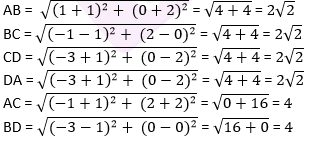Side length = AB = BC = CD = DA = 2√2

Diagonal Measure = AC = BD = 4

Therefore, the given points are the vertices of a square.

(ii) Let the points (- 3, 5), (3, 1), (0, 3), and ( – 1, – 4) be representing the vertices A, B, C, and D of the given quadrilateral respectively.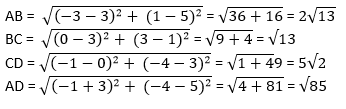All sides of this quadrilateral are of different lengths. Therefore, it can be said that it is a general quadrilateral.

(iii) Let the points (4, 5), (7, 6), (4, 3), and (1, 2) be representing the vertices A, B, C, and D of the given quadrilateral respectively.

Opposite sides of this quadrilateral are of the same length. However, the diagonals are of different lengths. Therefore, the given points are the vertices of a parallelogram.

7. Find the point on the x-axis which is equidistant from (2, – 5) and (- 2, 9).

Solution:

To find a point on x-axis. Therefore, its y-coordinate will be 0. Let the point on x-axis be (x,0).

Consider A = (x, 0); B = (2, – 5) and C = (- 2, 9).Simplify the above equation,

Remove square root by taking square both the sides, we get

(2-x)+ 25 = (-2-x)2+81

x2+4-4x+25 = x2+4+4x+81

8x = 25-81 = -56

x = -7

Therefore, the point is (- 7, 0).

8. Find the values of y for which the distance between the points P (2, – 3) and Q (10, y) is 10 units.

Solution:

Given: Distance between (2, – 3) and (10, y) is 10.

Using distance formula,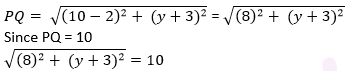Simplify the above equation and find the value of y.

Squaring both sides,

64 +(y+3)2 = 100

(y+3)= 36

y + 3 = ±6

y + 3 = +6 or y + 3 = −6

Therefore, y = 3 or -9.

9. If Q (0, 1) is equidistant from P (5, – 3) and R (x, 6), find the values of x. Also find the distance QR and PR.

Solution:

Given: Q (0, 1) is equidistant from P (5, – 3) and R (x, 6), which means PQ = QR

Step 1: Find the distance between PQ and QR using distance formula,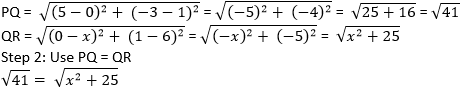Squaring both the sides, to omit square root

41 = x2+25

x= 16

x = ± 4

x = 4 or x = -4

Coordinates of Point R will be R (4, 6) OR R (-4, 6),

If R (4, 6), then QR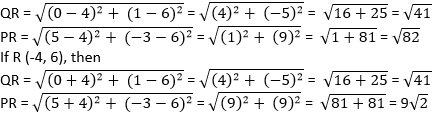10. Find a relation between x and y such that the point (x, y) is equidistant from the point (3, 6) and (- 3, 4).

Solution:

Point (x, y) is equidistant from (3, 6) and ( – 3, 4).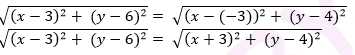Squaring both sides, (x-3)2+(y-6)2 = (x +3)2 +(y-4)2

x+9-6x+y2+36-12y = x+9+6x+y+16-8y

36-16 = 6x+6x+12y-8y

20 = 12x+4y

3x+y = 5

3x+y-5 = 0

## Exercise 7.2 Class 10 Maths NCERT Solution Page No: 167

1. Find the coordinates of the point which divides the join of (- 1, 7) and (4, – 3) in the ratio 2:3.

Solution:

Let P(xy) be the required point. Using the section formula, we get

x = (2×4+3×(-1))/(2+3) = (8-3)/5 = 1

y = (2×-3+3×7)/(2+3) = (-6+21)/5 = 3

Therefore, the point is (1, 3).

2. Find the coordinates of the points of trisection of the line segment joining (4, -1) and (-2, -3).

Solution: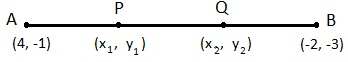Let P (x1, y1) and Q (x2, y2) are the points of trisection of the line segment joining the given points i.e., AP = PQ = QB

Therefore, point P divides AB internally in the ratio 1:2.

x= (1×(-2)+2×4)/3 = (-2+8)/3 = 6/3 = 2

y1 = (1×(-3)+2×(-1))/(1+2) = (-3-2)/3 = -5/3

Therefore: P (x1, y1) = P(2, -5/3)

Point Q divides AB internally in the ratio 2:1.

x2 = (2×(-2)+1×4)/(2+1) = (-4+4)/3 = 0

y2 = (2×(-3)+1×(-1))/(2+1) = (-6-1)/3 = -7/3

The coordinates of the point Q is (0, -7/3)

3. To conduct Sports Day activities, in your rectangular shaped school ground ABCD, lines have been drawn with chalk powder at a distance of 1 m each. 100 flower pots have been placed at a distance of 1 m from each other along AD, as shown in the following figure. Niharika runs 1/4 th the distance AD on the 2nd line and posts a green flag. Preet runs 1/5th the distance AD on the eighth line and posts a red flag. What is the distance between both the flags? If Rashmi has to post a blue flag exactly halfway between the line segment joining the two flags, where should she post her flag?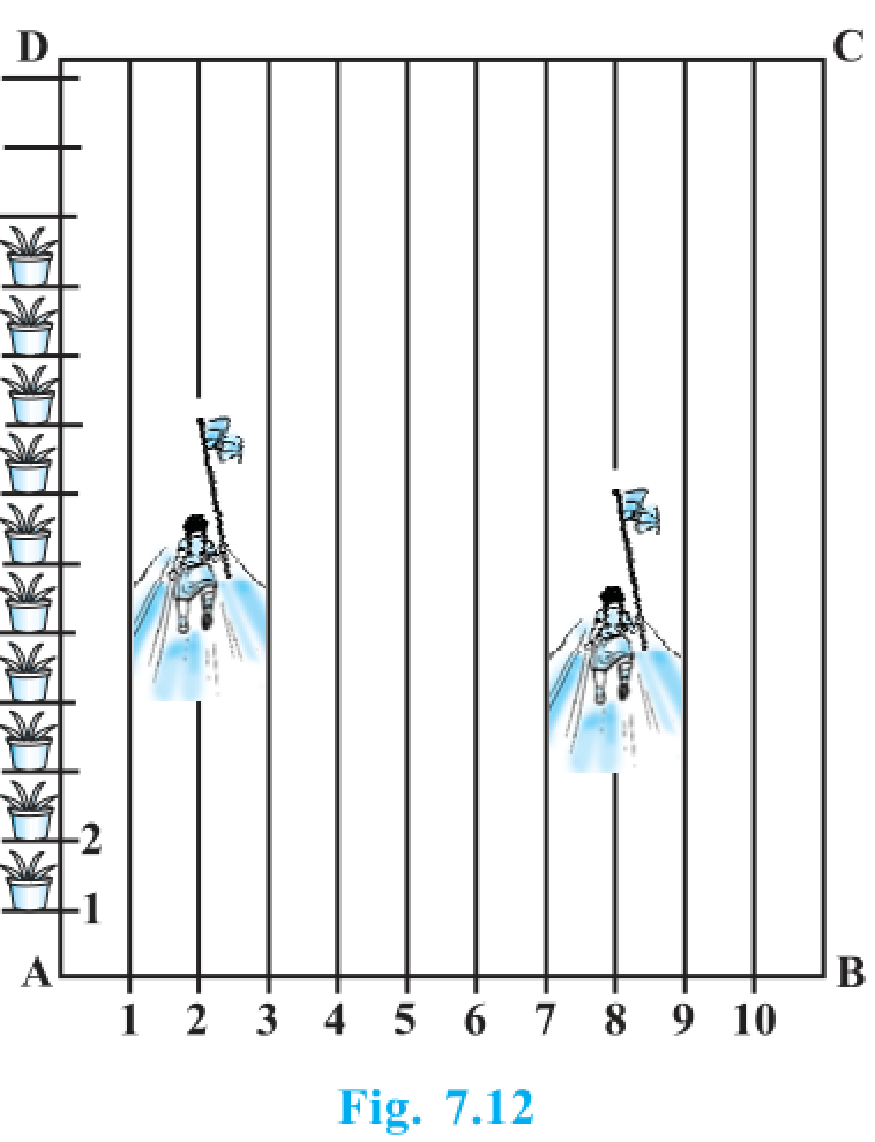Solution:

From the given instruction, we observed that Niharika posted the green flag at 1/4th of the distance AD i.e., (1/4 ×100) m = 25m from the starting point of 2nd line. Therefore, the coordinates of this point are (2, 25).

Similarly, Preet posted red flag at 1/5 of the distance AD i.e., (1/5 ×100) m = 20m from the starting point of 8th line. Therefore, the coordinates of this point are (8, 20).

Distance between these flags can be calculated by using distance formula,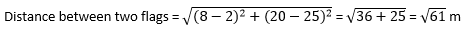The point at which Rashmi should post her blue flag is the mid- point of the line joining these points. Let say this point be P(x, y).

x = (2+8)/2 = 10/2 = 5 and y = (20+25)/2 = 45/2

Hence, P( xy) = (5,45/2)

Therefore, Rashmi should post her blue flag at 45/2 = 22.5m on 5th line.

4. Find the ratio in which the line segment joining the points (-3, 10) and (6, – 8) is divided by (-1, 6).

Solution:

Consider the ratio in which the line segment joining ( -3, 10) and (6, -8) is divided by point ( -1, 6) be k :1.

Therefore, -1 = ( 6k-3)/(k+1)

– 1 = 6-3

7= 2

= 2/7

Therefore, the required ratio is 2:7.

5. Find the ratio in which the line segment joining A (1, – 5) and B (- 4, 5) is divided by the x-axis. Also find the coordinates of the point of division.

Solution:

Let the ratio in which the line segment joining A (1, – 5) and B ( – 4, 5) is divided by x-axis be k : 1. Therefore, the coordinates of the point of division, say P(x, y) is ((-4k+1)/(k+1), (5k-5)/(k+1)).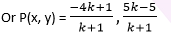We know that y-coordinate of any point on x-axis is 0.

Therefore, ( 5k-5)/(k+1) = 0

5k = 5

or k = 1

So, x-axis divides the line segment in the ratio 1:1.

Now, find the coordinates of the point of division:

P (x, y) = ((-4(1)+1)/(1+1) , (5(1)-5)/(1+1)) = (-3/2 , 0)

6. If (1, 2), (4, y), (x, 6) and (3, 5) are the vertices of a parallelogram taken in order, find and y.

Solution:

Let A,B,C and D be the points of a parallelogram : A(1,2), B(4,y), C(x,6) and D(3,5).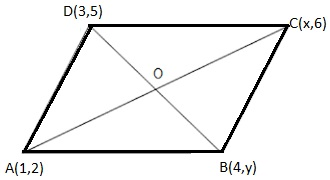Since the diagonals of a parallelogram bisect each other, the midpoint is same.

To find the value of x and y, solve for midpoint first.

Midpoint of AC = ( (1+x)/2 , (2+6)/2 ) = ((1+x)/2 , 4)

Midpoint of BD = ((4+3)/2 , (5+y)/2 ) = (7/2 , (5+y)/2)

Midpoint of AC and BD are same, this implies

(1+x)/2 = 7/2 and 4 = (5+y)/2

+ 1 = 7 and 5 + = 8

= 6 and = 3. Answer!

7. Find the coordinates of a point A, where AB is the diameter of circle whose centre is (2, – 3) and B is (1,4).

Solution:

Let the coordinates of point A be (xy).

Mid-point of AB is (2, – 3), which is the centre of the circle.

Coordinate of B = (1, 4)

(2, -3) =((x+1)/2 , (y+4)/2)

(x+1)/2 = 2 and (y+4)/2 = -3

x + 1 = 4 and y + 4 = -6

x = 3 and y = -10

8. If A and B are (-2, -2) and (2, -4), respectively, find the coordinates of P such that AP = 3/7 AB and P lies on the line segment AB.

Solution: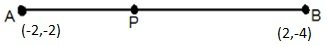The coordinates of point A and B are (-2,-2) and (2,-4) respectively. Since AP = 3/7 AB

Therefore, AP: PB = 3:4

Point P divides the line segment AB in the ratio 3:4.

9. Find the coordinates of the points which divide the line segment joining A (- 2, 2) and B (2, 8) into four equal parts.

Solution:

Draw a figure, line dividing by 4 points.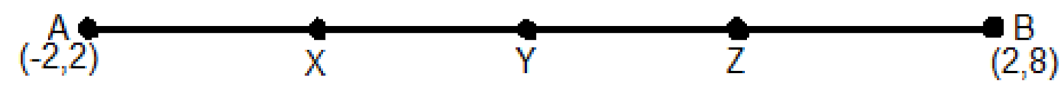From the figure, it can be observed that points X, Y, Z are dividing the line segment in a ratio 1:3, 1:1, 3:1 respectively.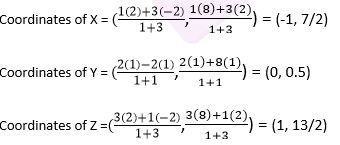10. Find the area of a rhombus if its vertices are (3, 0), (4, 5), (-1, 4) and (-2,-1) taken in order.

[Hint: Area of a rhombus = 1/2(product of its diagonals)

Solution:

Let A(3, 0), B (4, 5), C( – 1, 4) and D ( – 2, – 1) are the vertices of a rhombus ABCD.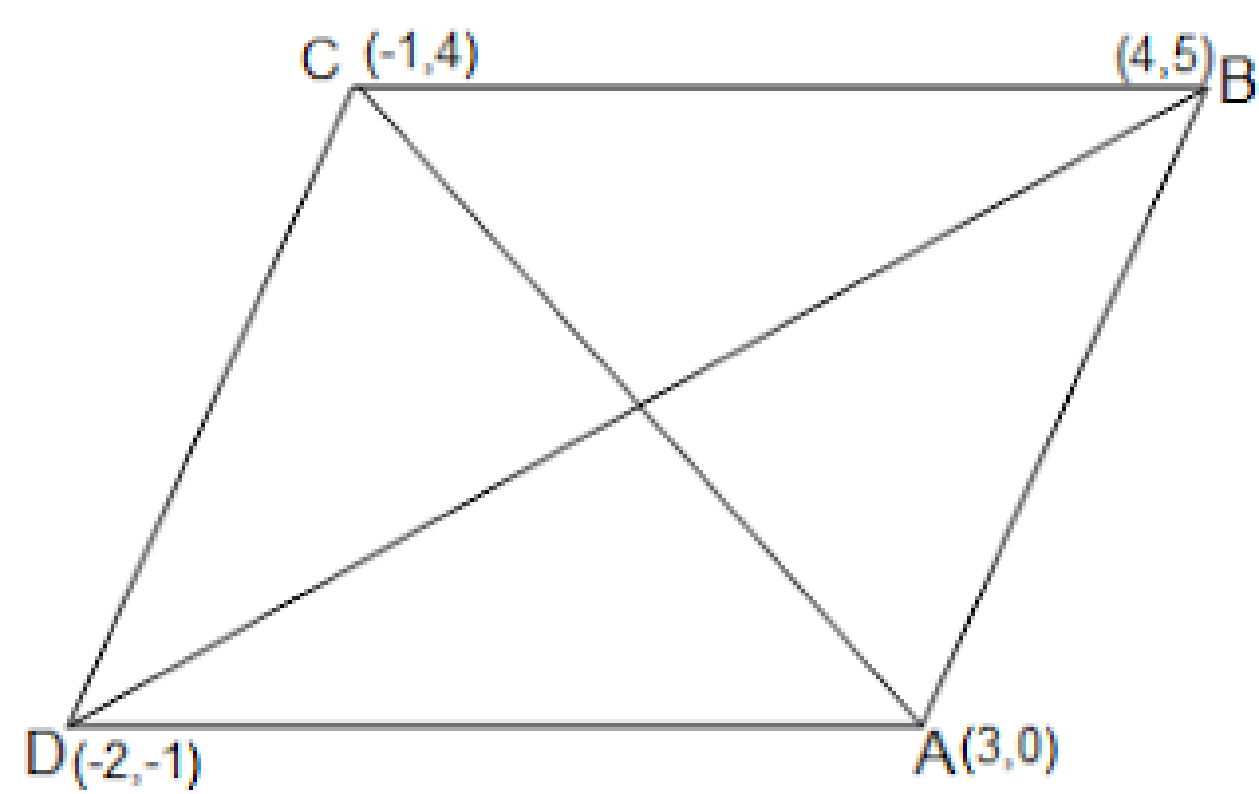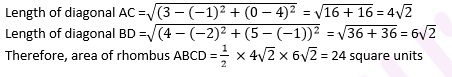## Exercise 7.3 Class 10 Maths NCERT Solution Page No: 170

1. Find the area of the triangle whose vertices are:

(i) (2, 3), (-1, 0), (2, -4)

(ii) (-5, -1), (3, -5), (5, 2)

Solution:

Area of a triangle formula =

(i) Here,

Substitute all the values in the above formula, we get

Area of triangle = 1/2 [2 {0- (-4)} + (-1) {(-4) – (3)} + 2 (3 – 0)]

= 1/2 {8 + 7 + 6} = 21/2

So area of triangle is 21/2 square units.

(ii) Here,

Area of the triangle = 1/2 [-5 { (-5)- (2)} + 3(2-(-1)) + 5{-1 – (-5)}]

= 1/2{35 + 9 + 20} = 32

Therefore, area of the triangle is 32 square units.

2. In each of the following find the value of ‘k’, for which the points are collinear.

(i) (7, -2), (5, 1), (3, -k)

(ii) (8, 1), (k, -4), (2, -5)

Solution:

(i) For collinear points, area of triangle formed by them is always zero.

Let points (7, -2) (5, 1), and (3, k) are vertices of a triangle.

Area of triangle = 1/2 [7 { 1- k} + 5(k-(-2)) + 3{(-2) – 1}] = 0

7 – 7k + 5k +10 -9 = 0

-2k + 8 = 0

k = 4

(ii) For collinear points, area of triangle formed by them is zero.

Therefore, for points (8, 1), (k, – 4), and (2, – 5), area = 0

1/2 [8 { -4- (-5)} + k{(-5)-(1)} + 2{1 -(-4)}] = 0

8 – 6k + 10 = 0

6k = 18

k = 3

3. Find the area of the triangle formed by joining the mid-points of the sides of the triangle whose vertices are (0, -1), (2, 1) and (0, 3). Find the ratio of this area to the area of the given triangle.

Solution:

Let the vertices of the triangle be A (0, -1), B (2, 1), C (0, 3).

Let D, E, F be the mid-points of the sides of this triangle.

Coordinates of D, E, and F are given by

D = ( , ) = (1, 0)

E = ( , ) = (0, 1)

F = ( , ) = (1, 2)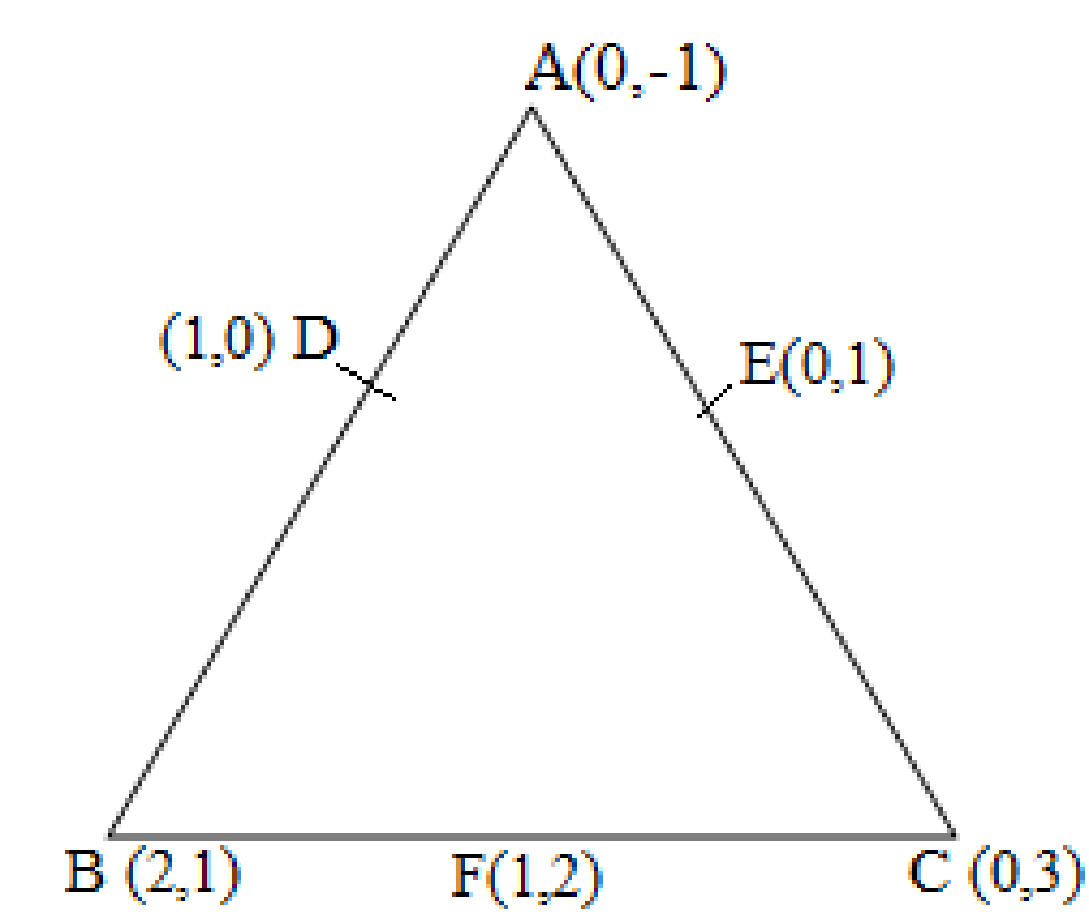Area of a triangle =

Area of ΔDEF = 1/2 {1(2-1) + 1(1-0) + 0(0-2)} = 1/2 (1+1) = 1

Area of ΔDEF is 1 square units

Area of ΔABC = 1/2 [0(1-3) + 2{3-(-1)} + 0(-1-1)] = 1/2 {8} = 4

Area of ΔABC is 4 square units

Therefore, the required ratio is 1:4.

4. Find the area of the quadrilateral whose vertices, taken in order, are

(-4, -2), (-3, -5), (3, -2) and (2, 3).

Solution:

Let the vertices of the quadrilateral be A (- 4, – 2), B ( – 3, – 5), C (3, – 2), and D (2, 3). Join AC and divide quadrilateral into two triangles.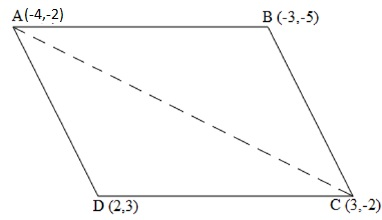We have two triangles ΔABC and ΔACD.

Area of a triangle =

Area of ΔABC = 1/2 [(-4) {(-5) – (-2)} + (-3) {(-2) – (-2)} + 3 {(-2) – (-5)}]

= 1/2 (12 + 0 + 9)

= 21/2 square units

Area of ΔACD = 1/2 [(-4) {(-2) – (3)} + 3{(3) – (-2)} + 2 {(-2) – (-2)}]

= 1/2 (20 + 15 + 0)

= 35/2 square units

Area of quadrilateral ABCD = Area of ΔABC + Area of ΔACD

= (21/2 + 35/2) square units = 28 square units

5. You have studied in Class IX that a median of a triangle divides it into two triangles of equal areas. Verify this result for ΔABC whose vertices are A (4, – 6), B (3, – 2) and C (5, 2).

Solution:

Let the vertices of the triangle be A (4, -6), B (3, -2), and C (5, 2).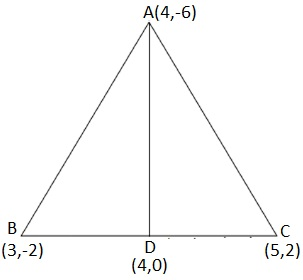Let D be the mid-point of side BC of ΔABC. Therefore, AD is the median in ΔABC.

Coordinates of point D = Midpoint of BC = ( = (4, 0)

Formula, to find Area of a triangle =

Now, Area of ΔABD = 1/2 [(4) {(-2) – (0)} + 3{(0) – (-6)} + (4) {(-6) – (-2)}]

= 1/2 (-8 + 18 – 16)

= -3 square units

However, area cannot be negative. Therefore, area of ΔABD is 3 square units.

Area of ΔACD = 1/2 [(4) {0 – (2)} + 4{(2) – (-6)} + (5) {(-6) – (0)}]

= 1/2 (-8 + 32 – 30) = -3 square units

However, area cannot be negative. Therefore, area of ΔACD is 3 square units.

The area of both sides is same. Thus, median AD has divided ΔABC in two triangles of equal areas.

## Exercise 7.4 Class 10 Maths NCERT Solution Page No: 171

1. Determine the ratio in which the line 2x + y – 4 = 0 divides the line segment joining the points A(2, –2) and B(3, 7).

Solution:

Consider line 2x + y – 4 = 0 divides line AB joined by the two points A(2, -2) and B(3, 7) in k : 1 ratio.

Coordinates of point of division can be given as follows:

x = and y =

Substituting the values of x and y given equation, i.e. 2x + y – 4 = 0, we have

2( + (– 4 = 0

+ ( = 4

4 + 6k – 2 + 7k = 4(k+1)

-2 + 9k = 0

Or k = 2/9

Hence, the ratio is 2:9.

2. Find the relation between x and y if the points (x, y), (1, 2) and (7, 0) are collinear.

Solution:

If given points are collinear then area of triangle formed by them must be zero.

Let (x, y), (1, 2) and (7, 0) are vertices of a triangle,

Area of a triangle = = 0

[x(2 – 0) + 1 (0 – y) + 7( y – 2)] = 0

• 2x – y + 7y – 14 = 0
• 2x + 6y – 14 = 0
• x + 3y – 7 = 0. Which is required result.

3. Find the centre of a circle passing through points (6, -6), (3, -7) and (3, 3).

Solution:

Let A = (6, -6), B = (3, -7), C = (3, 3) are the points on a circle.

If O is the centre, then OA = OB = OC (radii are equal)

If O = (x, y) then

OA =

OB =

OC =

Choose: OA = OB, we have

After simplifying above, we get -6x = 2y – 14 ….(1)

Similarly: OB = OC

(x – 3)+ (y + 7)2 = (x – 3)2 + (y – 3)2

(y + 7)2 = (y – 3)2

y+ 14y + 49 = y– 6y + 9

20y =-40

or y = -2

Substituting the value of y in equation (1), we get;

-6x = 2y – 14

-6x = -4 – 14 = -18

x = 3

Hence, centre of the circle located at point (3,-2).

4. The two opposite vertices of a square are (-1, 2) and (3, 2). Find the coordinates of the other two vertices.

Solution:

Let ABCD is a square, where A(-1,2) and B(3,2). And Point O is the point of intersection of AC and BD

To Find: Coordinate of points B and D.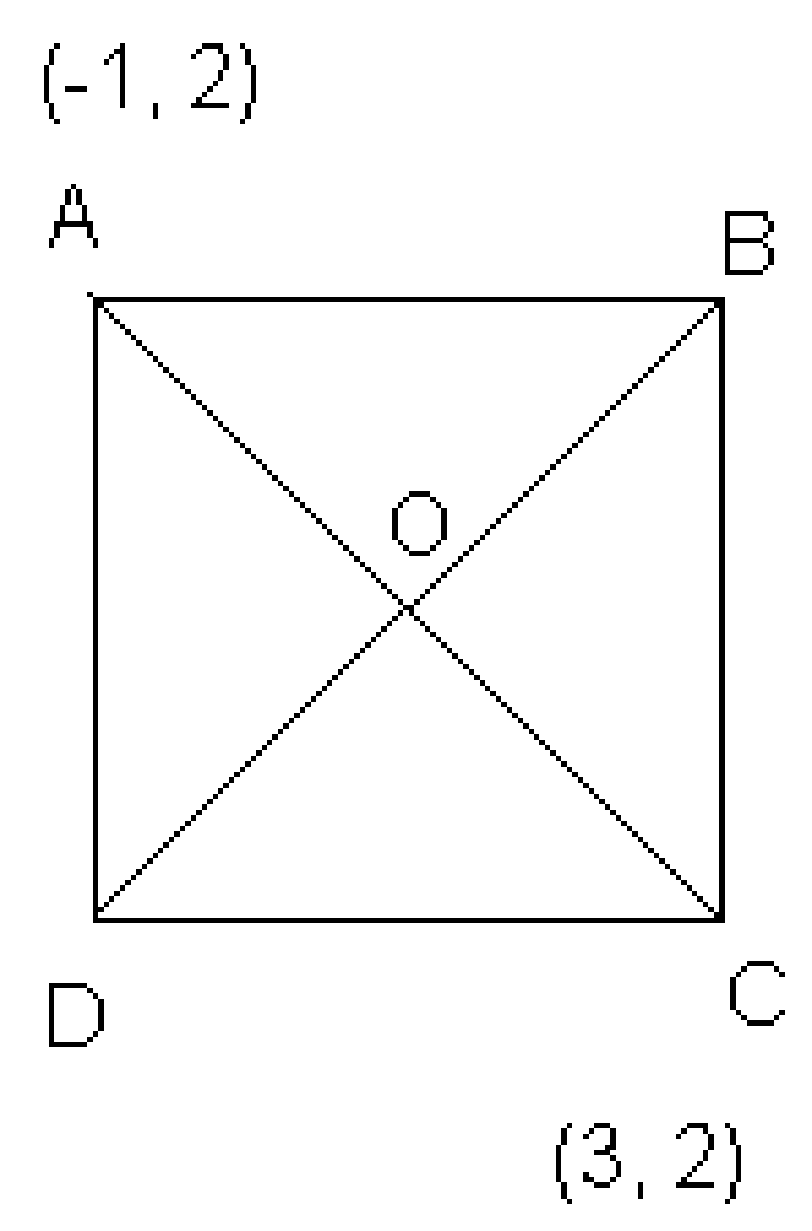Step 1: Find distance between A and C and coordinates of point O.

We know that, diagonals of a square are equal and bisect each other.

AC = = 4

Coordinates of O can be calculated as follows:

x = (3-1)/2 = 1 and y = (2+2)/2 = 2

So O(1,2)

Step 2: Find the side of the square using Pythagoras theorem

Let a be the side of square and AC = 4

From right triangle, ACD,

a = 2√2

Hence, each side of square = 2√2

Step 3: Find coordinates of point D

Equate length measure of AD and CD

Say, if coordinate of D are

Squaring both sides,

Similarly, CD2 =

Since all sides of a square are equal, which means AD = CD

Value of y1 can be calculated as follows by using the value of x.

From step 2: each side of square = 2√2

CD2 =

8 =

Hence, D = (1, 4)

Step 4: Find coordinates of point B

From line segment, BOD

Coordinates of B can be calculated using coordinates of O; as follows:

Earlier, we had calculated O = (1, 2)

Say B =

For BD;

1 =

x= 1

And 2 =

=> y2 = 0

Therefore, the coordinates of required points are B = (1,0) and D = (1,4)

5. The class X students of a secondary school in Krishinagar have been allotted a rectangular plot of land for their gardening activity. Saplings of Gulmohar are planted on the boundary at a distance of 1 m from each other. There is a triangular lawn in the plot as shown in the fig. 7.14. The students are to sow the seeds of flowering plants on the remaining area of the plot.

(i) Taking A as origin, find the coordinates of the vertices of the triangle.

(ii) What will be the coordinates of the vertices of triangle PQR if C is the origin?

Also calculate the areas of the triangles in these cases. What do you observe?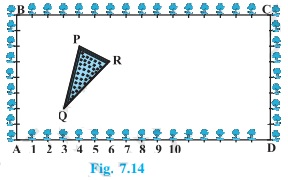Solution:

(i) Taking A as origin, coordinates of the vertices P, Q and R are,

From figure: P = (4, 6), Q = (3, 2), R (6, 5)

Here AD is the x-axis and AB is the y-axis.

(ii) Taking C as origin,

Coordinates of vertices P, Q and R are ( 12, 2) , (13, 6) and (10, 3) respectively.

Here CB is the x-axis and CD is the y-axis.

Find the area of triangles:

Area of triangle PQR in case of origin A:

Using formula: Area of a triangle =

= ½ [4(2 – 5) + 3 (5 – 6) + 6 (6 – 2)]

= ½ ( – 12 – 3 + 24 )

= 9/2 sq unit

(ii) Area of triangle PQR in case of origin C:

Area of a triangle =

= ½ [ 12(6 – 3) + 13 ( 3 – 2) + 10( 2 – 6)]

= ½ ( 36 + 13 – 40)

= 9/2 sq unit

This implies, Area of triangle PQR at origin A = Area of triangle PQR at origin C

Area is same in both case because triangle remains the same no matter which point is considered as origin.

6. The vertices of a ∆ ABC are A (4, 6), B (1, 5) and C (7, 2). A line is drawn to intersect sides AB and AC at D and E respectively, such that . Calculate the area of the ∆ ADE and compare it with area of ∆ ABC. (Recall Theorem 6.2 and Theorem 6.6)

Solution:

Given: The vertices of a ∆ ABC are A (4, 6), B (1, 5) and C (7, 2)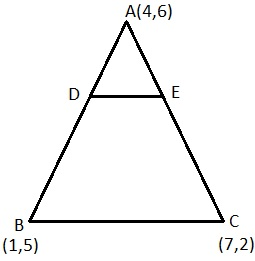Point D and Point E divide AB and AC respectively in ratio 1 : 3.

Coordinates of D can be calculated as follows:

x = and y =

Here

Consider line segment AB which is divided by the point D at the ration 1:3.

x =

y =

Similarly, Coordinates of E can be calculated as follows:

x =

y = = 5

Find Area of triangle:

Using formula: Area of a triangle =

Area of triangle ∆ ABC can be calculated as follows:

= ½ [4(5 – 2) + 1( 2 – 6) + 7( 6 – 5)]

= ½ (12 – 4 + 7) = 15/2 sq unit

Area of ∆ ADE can be calculated as follows:

= ½ [4(23/4 – 5) + 13/4 (5 – 6) + 19/4 (6 – 23/4)]

= ½ (3 – 13/4 + 19/16)

= ½ ( 15/16 ) = 15/32 sq unit

Hence, ratio of area of triangle ADE to area of triangle ABC = 1 : 16.

7. Let A (4, 2), B (6, 5) and C (1, 4) be the vertices of ∆ ABC.

(i) The median from A meets BC at D. Find the coordinates of point D.

(ii) Find the coordinates of the point P on AD such that AP : PD = 2 : 1.

(iii) Find the coordinates of point Q and R on medians BE and CF respectively such that BQ : QE = 2:1 and CR : RF = 2 : 1.

(iv) What do you observe?

[Note : The point which is common to all the three medians is called the centroid

and this point divides each median in the ratio 2 : 1.](v) If A (x1, y1), B (x2, y2) and C (x3, y3) are the vertices of triangle ABC, find the coordinates of the centroid of the triangle.

Solution: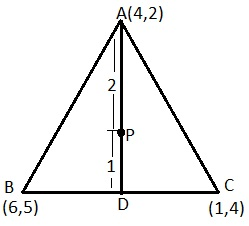(i) Coordinates of D can be calculated as follows:

Coordinates of D =

D

(ii) Coordinates of P can be calculated as follows:

Coordinates of P =

P

(iii) Coordinates of E can be calculated as follows:

Coordinates of E = = (5/2 , 3)

E(5/2 , 3)

Point Q and P would be coincident because medians of a triangle intersect each other at a common point called centroid. Coordinate of Q can be given as follows:

Coordinates of Q =

F is the mid- point of the side AB

Coordinates of F =

Point R divides the side CF in ratio 2:1

Coordinates of R =

(iv) Coordinates of P, Q and R are same which shows that medians intersect each other at a common point, i.e. centroid of the triangle.

(v) If A (x1, y1), B (x2, y2) and C (x3, y3) are the vertices of triangle ABC, the coordinates of centroid can be given as follows:

8. ABCD is a rectangle formed by the points A (-1, – 1), B (-1, 4), C (5, 4) and D (5, -1). P, Q, R and S are the midpoints of AB, BC, CD and DA respectively. Is the quadrilateral PQRS a square? a rectangle? or a rhombus? Justify your answer.

Solution: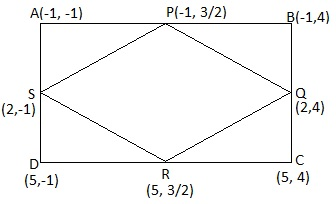P id the mid-point of side AB,

Coordinate of P =

Similarly, Q, R and S are (As Q is mid-point of BC, R is midpoint of CD and S is midpoint of AD)

Coordinate of Q = (2, 4)

Coordinate of R = (5,

Coordinate of S = (2, -1)

Now,

Length of PQ = = =

Length of SP = = =

Length of QR = = =

Length of RS = = =

Length of PR (diagonal) = = 6

Length of QS (diagonal) = = 5

The above values show that, PQ = SP = QR = RS = , i.e. all sides are equal.

But PR ≠ QS i.e. diagonals are not of equal measure.

Hence, the given figure is a rhombus.